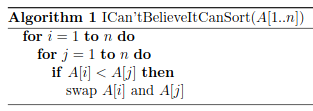# The “I Can't Believe It Can Sort” AlgorithmThe abstract: “We present an extremely simple sorting algorithm. It may look like it is obviously wrong, but we prove that it is in fact correct. We compare it with other simple sorting algorithms, and analyse some of its curious properties.” Here is the full text, “Is this the simplest (and most surprising) sorting algorithm ever?” [PDF].

1 Like

As counterintuitive as this seems at first, it’s readily apparent how it works. Of course, I had to immediately run out and try it — beautiful. I like the serendipitous nature of the author’s discovery.

He’s too modest in saying “There is nothing good about this algorithm.” Its beauty is in its simplicity and its surprise.

1 Like

I, too, had to try it for myself. If you’d like to, as well, here’s the algorithm in Perl.

```    my @A;
my \$n = 100;

#   Fill array with pseudorandom integers
for \$i (1 .. \$n) {
\$A[\$i] = int(rand(1000));
}

#   Algorithm 1 ICan'tBelieveItCanSort(A[1..n])
for \$i (1 .. \$n) {
for \$j (1 .. \$n) {
if (\$A[\$i] < \$A[\$j]) {
(\$A[\$j], \$A[\$i]) = (\$A[\$i], \$A[\$j]);
}
}
}

use Data::Dumper;
print(Dumper(\@A));
```

Lab rat that I am, I use Igor, which has a C-like programming language. The loop execution times vary according to how the random numbers fall: about 17 seconds for 1E4 numbers on my laptop.

``````Function sorting(numdata)
Variable numdata
Variable j,k,temp1,timeRefnum,microsecs
Make/O/N=(numdata)/D A
A=enoise(1)			// fill the array with pseudorandom reals
timeRefnum=StartMSTimer		//start the timer
Do
k=0
Do
If (A[j]<A[k])
temp1=A(j)
A[j]=A[k]
A[k]=temp1
else
endif
k+=1
While (k<numdata)
j+=1
While (j<numdata)
microsecs=StopMSTimer(timeRefnum)
Print microsecs/1e6,"seconds"
Display A
End
``````
1 Like

Here is my more concise, and allegedly more idiomatic, version:

``````my \$n = 10;
my @A = map int(rand(1000)), 1..\$n; # array of pseudorandom integers
#   Algorithm 1 ICan'tBelieveItCanSort(A[1..n])
for my \$i (0 .. \$#A) {
for my \$j (0 .. \$#A) {
@A[\$j,\$i] = @A[\$i,\$j] if \$A[\$i] < \$A[\$j];
}
}
use Data::Dumper;
print(Dumper(\@A));
``````